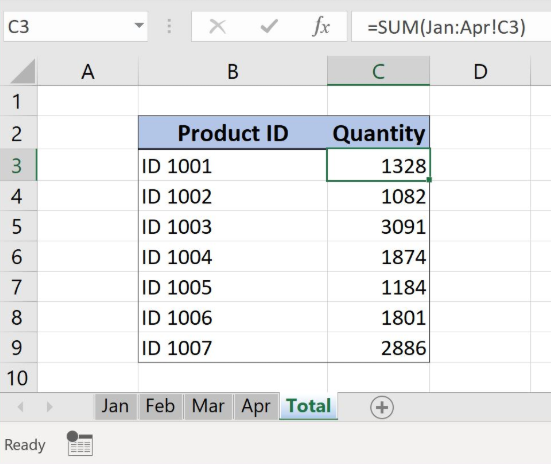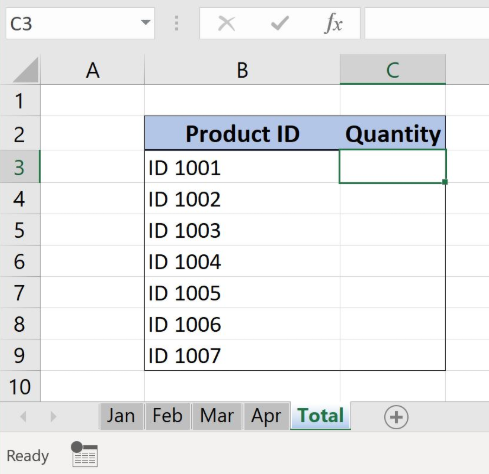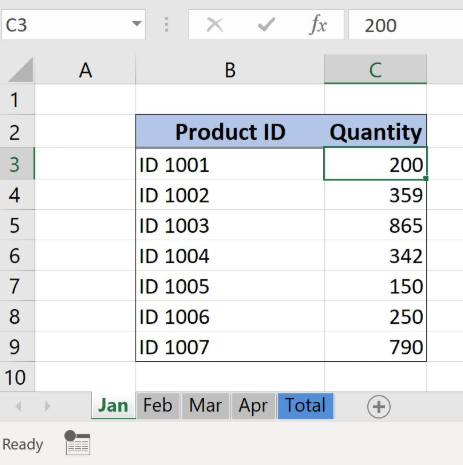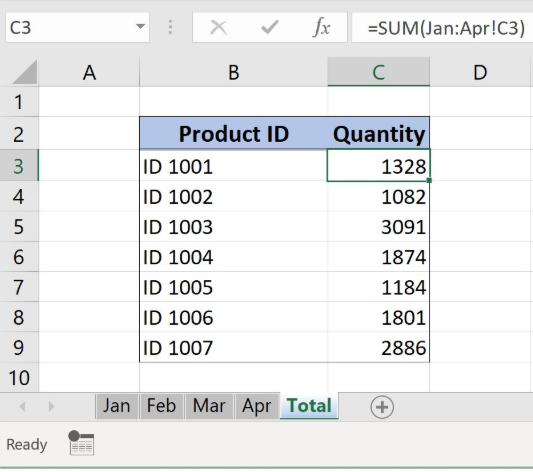Get instant live expert help with Excel or Google Sheets“My Excelchat expert helped me in less than 20 minutes, saving me what would have been 5 hours of work!”

#### Post your problem and you’ll get Expert help in seconds.

Your message must be at least 40 characters
Our professional Expert are available now. Your privacy is guaranteed.

# 3D Sum Multiple Worksheets

Excel allows us to sum the same cell ranges on the multiple worksheets by using SUM function with 3D reference syntax in the formula. This step by step tutorial will assist all levels of Excel users in summarizing the values in the same cell position on the several worksheets.Figure 1. 3D Sum Multiple Worksheets

## Syntax of the SUM Formula for Multiple Worksheets

`=SUM(first_sheet:last_sheet!cell)`

The parameters of the SUM function are:

• first_sheet – a first sheet name for summarizing the data
• last_sheet – the last sheet name for summarizing the data
• cell – cell or cell range reference from which we want to sum the values

## Setting up Our Data for the 3D SUM Function

Our table in sheet “Total” consists of 2 columns: “Product ID” (column B) and “Quantity” (column C).Figure 2. Table structure for 3D SUM example

The same table structure is in the same place in all sheets: “Jan”, “Feb”, “Mar” and “Apr”. The idea is to summarize the “Quantity” data per “Product ID” from all monthly sheets. The result will be presented in “Total” sheet in column “Quantity”.Figure 3. Monthly data that we will use in the 3D SUM example

## Summarize the Data from the Multiple Worksheets

We want to summarize the “Quantity” data per “Product ID” from sheets: “Jan”, “Feb”, “Mar” and “Apr”. For this, we will use the SUM function with the 3D reference because the structure and the position of the data are the same in all monthly sheets.

The formula looks like:

`=SUM(Jan:Apr!C3)`

The first_sheet is “Jan”, while the last_sheet is “Apr”. The cell reference from which we want to summarize the data is in the cell C3.

To apply the SUM function with 3D reference, we need to follow these steps:

• Select cell C3 in the sheet “Total” and click on it
• Insert sign “=” and type function SUM with open bracket “ =SUM( “
• Select the first monthly sheet with the data “Jan”
• Hold the Shift button and select the last monthly sheet “Apr” and cell C3
• Press enter
• Drag the formula down to the other cells in the column by clicking and dragging the little “+” icon at the bottom-right of the cell.Figure 4. Using the SUM function with 3D reference for summarizing the data from multiple sheets

Formula summarizes the values from the first_sheet to the last_sheet in the defined cell range. To include a new sheet in a sum range we just have to place it somewhere between the first and the last sheet from the formula. To use 3D reference in SUM function it’s important to have the same data source structure and the position.

Most of the time, the problem you will need to solve will be more complex than a simple application of a formula or function. If you want to save hours of research and frustration, try our live Excelchat service! Our Excel Experts are available 24/7 to answer any Excel question you may have. We guarantee a connection within 30 seconds and a customized solution within 20 minutes.

### Did this post not answer your question? Get a solution from connecting with the expert.Another blog reader asked this question today on Excelchat:
Solution examplesI need a formula to pop at the upper left corner of a spreadsheet. If I enter the month "January," I want the column number sum of January =SUM(AB11:AB75) from another section on the same excel page to pop right below the "January" cell, and not display the formula expression, but see the \$100.
Solved by T. Q. in 40 minsCan't add (SUM) in imported numbers from bank account
Solved by F. C. in 40 minsI need a formula to combine D2 to D100 to add together a column of numbers, then take away the same amount on the same row when column E is filled. i.e. column D is a price of an item, so the formula must calculate the total, then when the item is sold an 'a' is marked next to the item in column E, the formula then must deduct this amount from the total
Solved by X. W. in 20 minsI would like to have a diagram in a new sheet, where the horizontal axis is the days, as they are in column DX. Each day shall show the sum of all unique leads of that day, and I would like to be able to check via a box of checkboxes, which facilities are shown, the facilities are in column BC.
Solved by I. A. in 45 minsI am working on a cash flow projection. Part of the projection includes sales commissions. Our sales guys earn a monthly draw and then commission on sales after a certain amount. For example they may earn a monthly salary of 12,500 and earn additional commission after their commission equals \$150,000. What I need excel to do is sum a column if the values in the preceeding columns are greater than \$150,000. I've tried using the sumif and the if function in excel and it's not working correctly either way. Any suggestions?
Solved by G. W. in 19 mins## Subscribe to Excelchat.coAnother blog reader asked this question today on Excelchat: